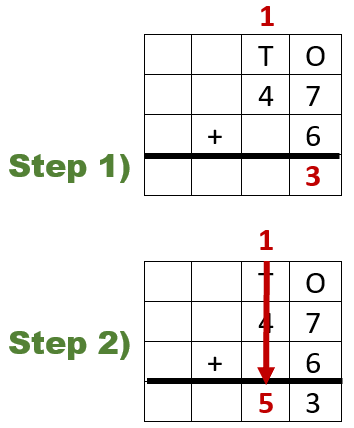# Math Worksheets Land

Math Worksheets For All Ages

# Math Worksheets Land

Math Worksheets For All Ages

Home > Math Topics > Addition >

# Adding Single and Double Digits Worksheets

This is one of those skills that you can learn to do mentally. There are basically three approaches teachers use to help students with this skill. The visual approach works great for students that have not mastered their basic math facts. It does help students to use little tens and ones place blocks. We would replicate the problem with those blocks and then have them draw out a final sum that is just a collection of those blocks. When you are working with students that have a good handle on their addition facts, have them learn to add the ones place and then the tens place. A nice way to combine both of these approaches is to get students to get in the habit of rewriting the problem vertically, if it is not provided. These worksheets and lessons help students master finding simple sum problems. Like anything the more exercises you do with your students, the faster they will understand it and retain it longer.

### Aligned Standard: 2.NBT.B.5

• Answer Keys - These are for all the unlocked materials above.

### Homework Sheets

It's always nice to start with an example problem completed for you.

• Homework 1 - We visually show you how to carry that value to the next place value.
• Homework 2 - Lets show you how to make 22. Then you get a 10 problem workout.
• Homework 3 - Some require carrying, but most don't.

### Practice Worksheets

These are peppered with tips and hint for students.

• Practice 1 - When you add numbers and it leads to a value of 10 or more, make sure to carry the one to the tens place.
• Practice 2 - A two-digit number has two place values.
• Practice 3 - The column on the left is called the tens place or tens column. Each number in this column has a value of ten. I.e. 5 is equal to 50 in the tens place.

### Math Skill Quizzes

Yeah, this is one of the few section where I added clip art to quizzes. I normally feel the need to make assessments more sterile, I guess.

• Quiz 1 - The column on the right is called the ones place or ones column. Each number has the value of 1.
• Quiz 2 - These are a bit bottom heavy. There will be a good bit of carrying values here.
• Quiz 3 - A nice selection of 3s, 4s, and 5s in this one.

### Tips to Learn to Add Single and Double DigitsWe can also apply a similar technique when we add two-digit numbers, we can break them apart by place values to get the answer. For instance, if we take an example of 13 added to 87, let's add them breaking them both. We will separate 13 into 10 and 3, while we will separate 80 and 7. We will add 3 and 7 from both numbers to make it 10. We will then add 10 and 80 to make 90. Then, we will add 90 and 10 to make the final answer 10. This technique can help us when we do not have any space to stretch out and write the problem vertically.

The best way to add a single digit to double digit value is to align them vertically and add by place value starting from the lowest available place value. Take a look at we have setup to the right (47 + 6). In the first step we add the lowest place value (ones column). When we add this column (7 + 6), we get a value that is a double-digit value (13). We take the 3 (ones place) from that sum and drop it as part of the sum for the ones place. We then carry the 1 (from 13) and add it to the tens place. We then calculate the value of the tens place (4 + 1 = 5). We drop the 5 in the tens place and our final sum is 53.

If we keep the following tips in mind, then adding numbers could become quite easy.

Unlock all the answers, worksheets, homework, tests and more!
Save Tons of Time! Make My Life Easier Now

## Thanks and Don't Forget To Tell Your Friends!

I would appreciate everyone letting me know if you find any errors. I'm getting a little older these days and my eyes are going. Please contact me, to let me know. I'll fix it ASAP.